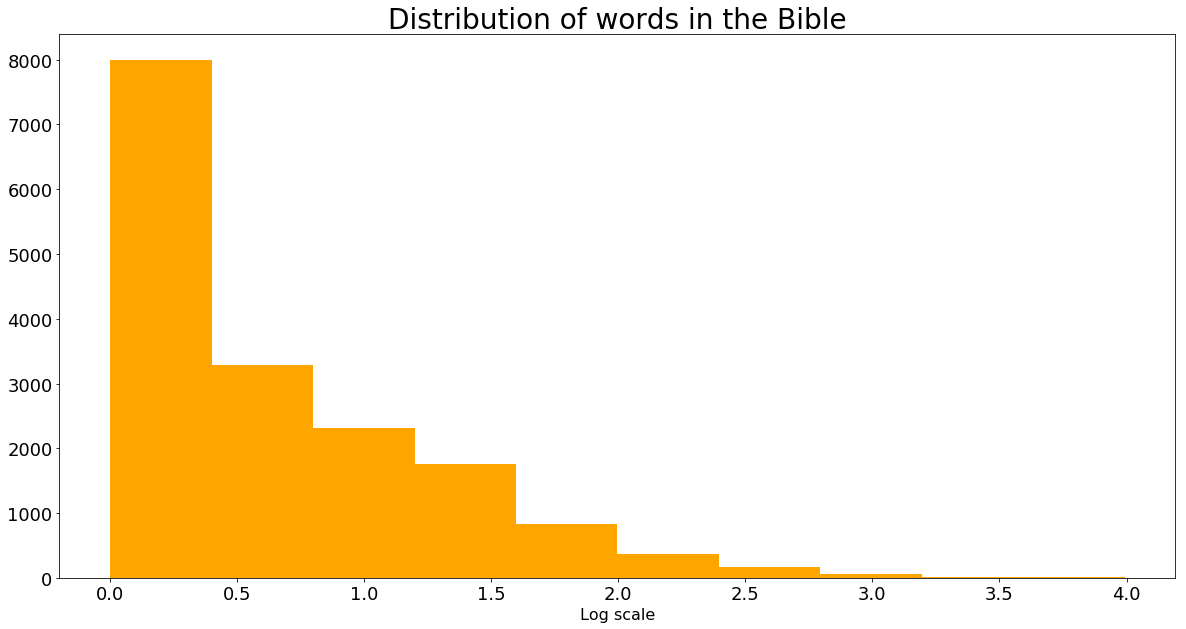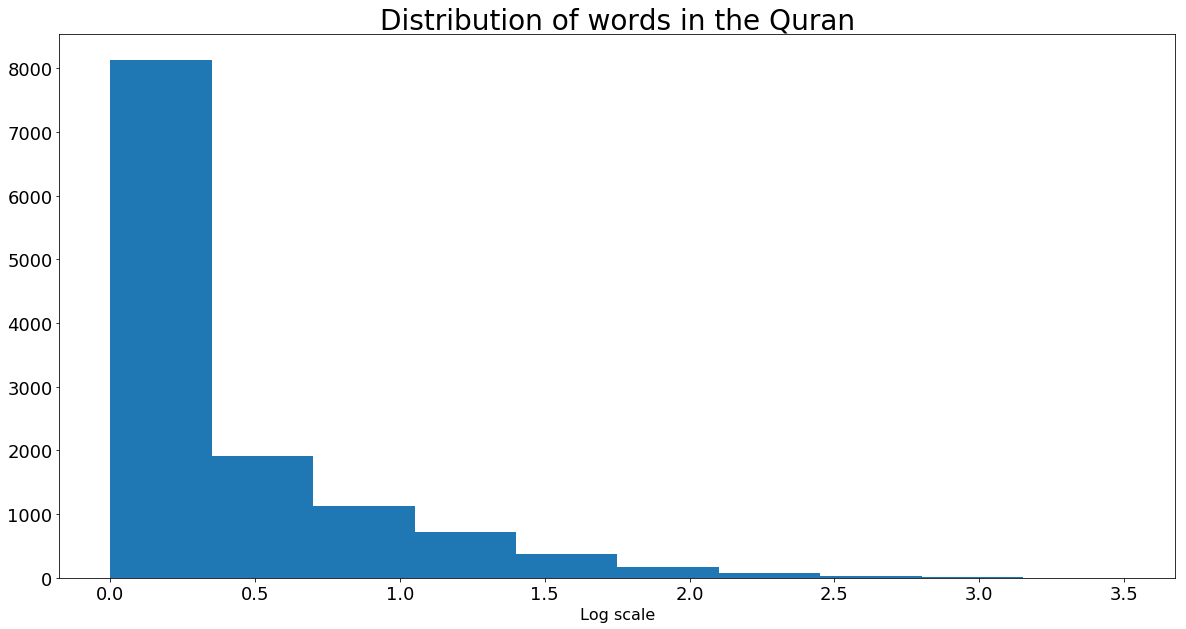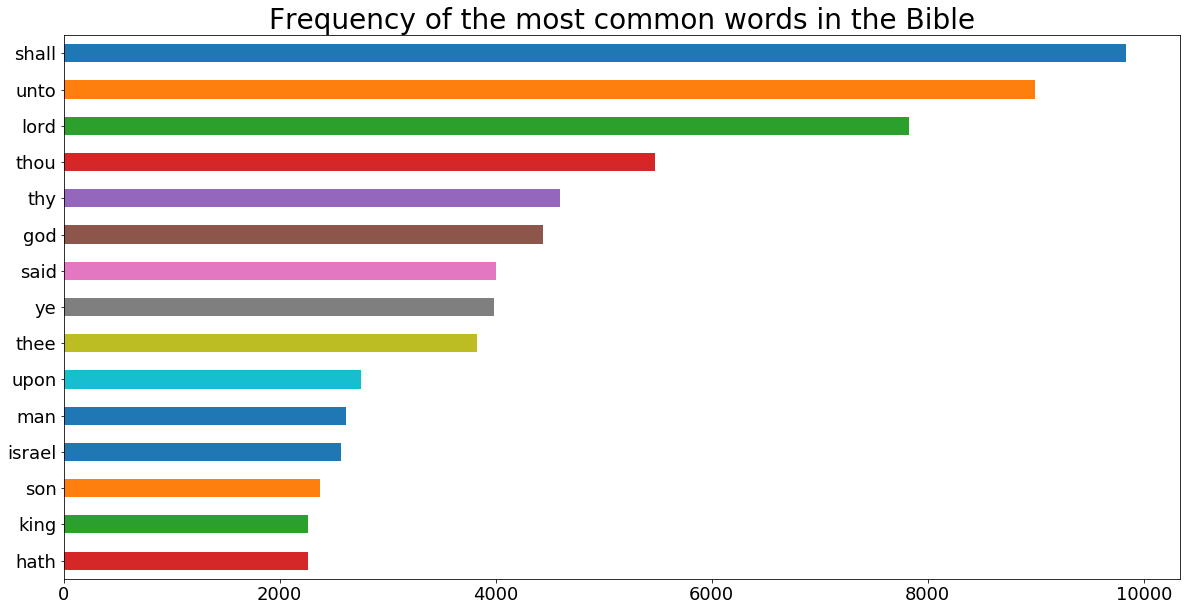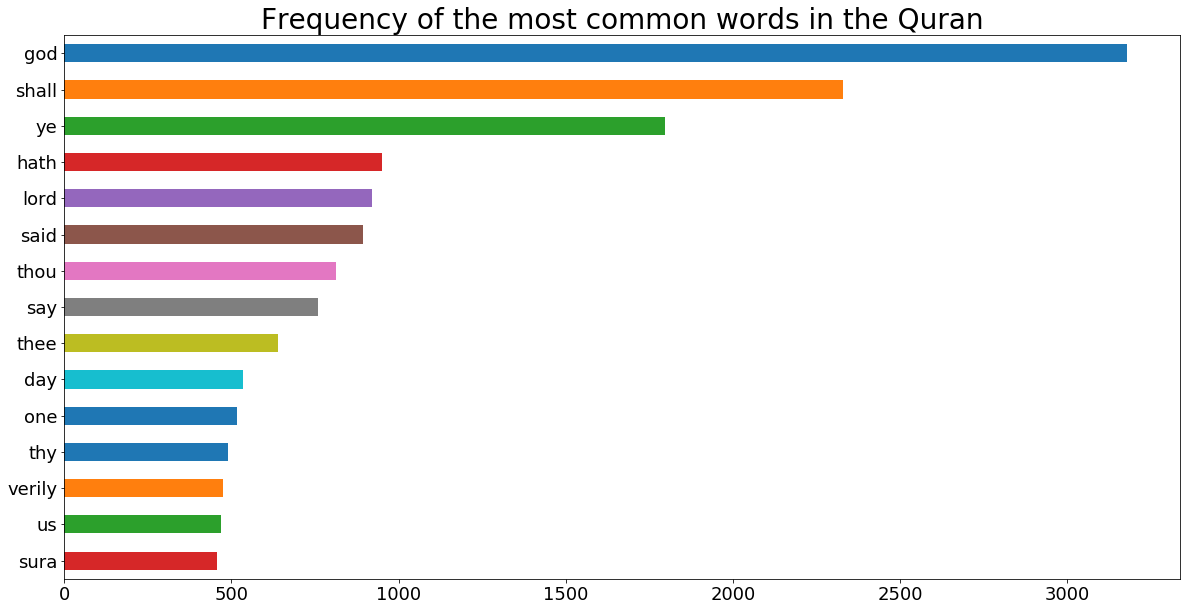# Fisseha Berhane, PhD

### Analyzing the Bible and the Quran using Spark¶

Most of the data out there is unstructured, and Spark is an excellent tool for analyzing this type of data. Here, we will analyze the Bible and the Quran. We will see the distribution of words, the most common words in both scriptures and the average frequency. This could also be scaled to find the most common words and distribution of all words on the Internet. The books have been retrieved from Project Gutenberg. The Bible can be downloaded from here and the Quran from here.

#### Import pyspark and initialize Spark¶

pyspark is the Spark Python API that exposes the Spark programming model to Python. SparkContext is main entry point for Spark functionality. In Spark, communication occurs between a driver and executors. The driver has Spark jobs that it needs to run and these jobs are split into tasks that are submitted to the executors for completion. The results from these tasks are delivered back to the driver. In order to use Spark and its API we will need to use a SparkContext. When running Spark, you start a new Spark application by creating a SparkContext. When the SparkContext is created, it asks the master for some cores to use to do work. The master sets these cores aside just for you; they won't be used for other applications. In the code below, we are also specifying some configuration parameters, including the fact that this Spark session is to use local machine since I am using my PC for this tutorial.

In :
from pyspark import SparkContext, SparkConf

conf = SparkConf().setAppName("miniProject").setMaster("local[*]")
sc = SparkContext.getOrCreate(conf)

##### Create Resilient Distributed Datasets (RDDs)¶

A Spark context can be used to create Resilient Distributed Datasets (RDDs) on a cluster.

To convert a text file into an RDD, we use the SparkContext.textFile() method.

In :
bibleRDD = sc.textFile("bible.txt")
quranRDD = sc.textFile("quran.txt")


#### Display sample data¶

collect return a list that contains all of the elements in the RDD.

In :
bibleRDD.sample(withReplacement = False, fraction = 0.0002, seed = 80).collect()

Out:
['will not do it, if I find thirty there.',
'work in the tabernacle of the congregation: 4:36 And those that were',
'Israel, which they bring unto the priest, shall be his.',
'',
'under mine hand, but there is hallowed bread; if the young men have',
'',
'',
'the king, All that thou didst send for to thy servant at the first I',
'5:7 And it came to pass, when the king of Israel had read the letter,',
'his clothes, and covered himself with sackcloth, and went into the',
'flowers, and the lamps, and the tongs, made he of gold, and that',
'',
'which they commit here? for they have filled the land with violence,',
'the house of Israel, to do it for them; I will increase them with men',
'whole limit thereof round about shall be most holy. Behold, this is',
'',
'Father which is in heaven.',
'21:31 Whether of them twain did the will of his father? They say unto',
'which were early at the sepulchre; 24:23 And when they found not his',
'peace.',
'red dragon, having seven heads and ten horns, and seven crowns upon',
'every man according to their works.']
In :
quranRDD.sample(withReplacement = False, fraction = 0.0002, seed = 80).collect()

Out:
['Many of them suffered torture for their faith in him, and two of them died as',
'when ye halt; and from their wool and soft fur and hair, hath He supplied you',
'meet for their best deeds.',
'',
'']

Let's count the number of lines in each RDD.

In :
print('The number of lines in the Bible text file is {}'.format(bibleRDD.count()))

The number of lines in the Bible text file is 100223

In :
print('The number of lines in the Quran text file is {}'.format(quranRDD.count()))

The number of lines in the Quran text file is 27321


Words should be counted independent of their capitialization. So, we will change all words to lower case. We will also remove all punctuations. Further, any leading or trailing spaces on a line should be removed.

The function below removes all characters which are not alpha-numeric except space(s). It also changes them to lower letter and removes leading or trailing spaces. As you can see we are using the python module re.

In :
import re

def wordclean(x):
return re.sub("[^a-zA-Z0-9\s]+","", x).lower().strip()


Let's check the above function:

In :
x = [" The Sun rises in the East and sets in the West!\n "
"  He said, 'I am sure you know the answer!\n'  "]

for i in x:
print(wordclean(i))

the sun rises in the east and sets in the west
he said i am sure you know the answer


Now, can apply it to our Bible and Quran RDDS. We use the map RDD method.

In :
bibleRDDList = bibleRDD.map(lambda x : wordclean(x))
quranRDDList = quranRDD.map(lambda x : wordclean(x))


Now, let's see how the RDDList files above look like. As shown below, all punctuation have been removed and all letters are lower-case.

In :
bibleRDDList.take(60)[41: ]

Out:
['11 in the beginning god created the heavens and the earth',
'',
'12 and the earth was without form and void and darkness was upon',
'the face of the deep and the spirit of god moved upon the face of the',
'waters',
'',
'13 and god said let there be light and there was light',
'',
'14 and god saw the light that it was good and god divided the light',
'from the darkness',
'',
'15 and god called the light day and the darkness he called night',
'and the evening and the morning were the first day',
'',
'16 and god said let there be a firmament in the midst of the waters',
'and let it divide the waters from the waters',
'',
'17 and god made the firmament and divided the waters which were',
'under the firmament from the waters which were above the firmament']
In :
quranRDDList.take(450)[414 : ]

Out:
['mohammed was born at mecca in ad 567 or 569  his flight hijra to medina',
'which marks the beginning of the mohammedan era took place on 16th june 622',
'he died on 7th june 632',
'',
'',
'',
'introduction',
'',
'the koran admittedly occupies an important position among the great religious',
'books of the world though the youngest of the epochmaking works belonging',
'to this class of literature it yields to hardly any in the wonderful effect',
'which it has produced on large masses of men it has created an all but new',
'phase of human thought and a fresh type of character it first transformed a',
'number of heterogeneous desert tribes of the arabian peninsula into a nation',
'of heroes and then proceeded to create the vast politicoreligious',
'organisations of the muhammedan world which are one of the great forces with',
'which europe and the east have to reckon today',
'',
'the secret of the power exercised by the book of course lay in the mind',
'which produced it it was in fact at first not a book but a strong living',
'voice a kind of wild authoritative proclamation a series of admonitions',
'promises threats and instructions addressed to turbulent and largely',
'hostile assemblies of untutored arabs as a book it was published after the',
'prophets death in muhammeds lifetime there were only disjointed notes',
'speeches and the retentive memories of those who listened to them to speak',
'of the koran is therefore practically the same as speaking of muhammed and',
'in trying to appraise the religious value of the book one is at the same time',
'attempting to form an opinion of the prophet himself it would indeed be',
'difficult to find another case in which there is such a complete identity',
'between the literary work and the mind of the man who produced it',
'',
'that widely different estimates have been formed of muhammed is wellknown',
'to moslems he is of course the prophet par excellence and the koran is',
'regarded by the orthodox as nothing less than the eternal utterance of allah',
'the eulogy pronounced by carlyle on muhammed in heroes and hero worship will',
'probably be endorsed by not a few at the present day the extreme contrary']

Apply a transformation that will split each element of the RDD by its spaces. For each element of the RDD, we are appling Python's string split() function. Note, we are using the flatMap here.

In :
bibleRDDwords = bibleRDDList.flatMap( lambda x: x.split(" "))
quranRDDwords = quranRDDList.flatMap( lambda x: x.split(" "))


Let's show sample words from each RDD.

In :
bibleRDDwords.sample(withReplacement = False, fraction = 0.00001, seed = 90).collect()

Out:
['isaac', 'even', 'amon', 'a', 'there', 'his', 'by', 'but']
In :
quranRDDwords.sample(withReplacement = False, fraction = 0.00005, seed = 90).collect()

Out:
['by', 'who', 'we', 'see', 'righteous', 'loveth', 'and', 'after', 'what', 'be']

Now, let's remove spaces. We use the filter method to achieve this.

In :
bibleRDDwords = bibleRDDwords.filter(lambda x: len(x) != 0)
quranRDDwords = quranRDDwords.filter(lambda x: len(x) != 0)


Next, let's create word pairs. This helps us to count the frequency of each word and to select the most common words in each RDD.

In :
bibleRDDwordPairs =  bibleRDDwords.map(lambda x: (x,1))
quranRDDwordPairs =  quranRDDwords.map(lambda x: (x, 1))


Let's show the first ten elements of each RDD.

In :
bibleRDDwordPairs.take(10)

Out:
[('the', 1),
('project', 1),
('gutenberg', 1),
('ebook', 1),
('of', 1),
('the', 1),
('king', 1),
('james', 1),
('bible', 1),
('this', 1)]
In :
quranRDDwordPairs.take(10)

Out:
[('the', 1),
('project', 1),
('gutenberg', 1),
('etext', 1),
('of', 1),
('the', 1),
('koran', 1),
('as', 1),
('translated', 1),
('by', 1)]

Now, we can find the frequency of each word.

The reduceByKey() transformation gathers together pairs that have the same key and applies a function to two associated values at a time. reduceByKey() operates by applying the function first within each partition on a per-key basis and then across the partitions.

In :
bibleRDDwordCount =  bibleRDDwordPairs.reduceByKey(lambda a, b : a + b)
quranRDDwordCount =  quranRDDwordPairs.reduceByKey(lambda a, b : a + b)

In :
bibleRDDwordCount.take(10)

Out:
[('admired', 1),
('11973', 1),
('shedeur', 5),
('stirs', 1),
('dispossessed', 2),
('tochen', 1),
('4833', 2),
('peor', 4),
('unblameable', 2),
('divers', 37)]
In :
quranRDDwordCount.take(10)

Out:
[('carious', 1),
('heedful', 1),
('lxiii1the', 1),
('combats', 1),
('denunciations', 2),
('calamitous', 1),
('divers', 3),
('afford', 2),
('weeks', 1),
('tents', 3)]

The takeOrdered() action returns the first n elements of the RDD, using either their natural order or a custom comparator. The key advantage of using takeOrdered() instead of first() or take() is that takeOrdered() returns a deterministic result, while the other two actions may return differing results, depending on the number of partions or execution environment. takeOrdered() returns the list sorted in ascending order. Note below, we are using -x to make it in descending order.

In :
bibleRDDwordCount.takeOrdered(10, lambda x : -x)

Out:
[('shall', 9840),
('unto', 8997),
('lord', 7830),
('thou', 5474),
('thy', 4600),
('god', 4442),
('said', 3999),
('ye', 3983),
('thee', 3826),
('upon', 2750)]
In :
quranRDDwordCount.takeOrdered(10, lambda x : -x)

Out:
[('god', 3180),
('shall', 2331),
('ye', 1798),
('hath', 951),
('lord', 921),
('said', 894),
('thou', 813),
('say', 758),
('thee', 640),
('day', 535)]

Next, let's remove stop words from our RDDs. Note that all old English stop words may not be included in the list of python stop words we are using here.

In :
import nltk

[nltk_data] Downloading package stopwords to /home/fish/nltk_data...
[nltk_data]   Package stopwords is already up-to-date!

Out:
True
In :
from nltk.corpus import stopwords

In :
stopwords = stopwords.words('english')

In :
len(stopwords)

Out:
153
In :
stopwords[0:10]

Out:
['i', 'me', 'my', 'myself', 'we', 'our', 'ours', 'ourselves', 'you', 'your']
In :
bibleRDDwordCount = bibleRDDwordCount.filter(lambda x : x not in stopwords)
quranRDDwordCount = quranRDDwordCount.filter(lambda x : x not in stopwords)


Now, we see the most frequent words from each RDD after removing the stop words. As shown below, God is the most frequent word in the Quran but sixth most frequent word in the Bible. In the bible, the word lord, which usually means God, is third most frequent word. Lord is fifth most common word in the Quran.

In :
bibleRDDwordCount.takeOrdered(10, lambda x : -x)

Out:
[('shall', 9840),
('unto', 8997),
('lord', 7830),
('thou', 5474),
('thy', 4600),
('god', 4442),
('said', 3999),
('ye', 3983),
('thee', 3826),
('upon', 2750)]
In :
quranRDDwordCount.takeOrdered(15, lambda x : -x)

Out:
[('god', 3180),
('shall', 2331),
('ye', 1798),
('hath', 951),
('lord', 921),
('said', 894),
('thou', 813),
('say', 758),
('thee', 640),
('day', 535),
('one', 517),
('thy', 489),
('verily', 475),
('us', 468),
('sura', 458)]

But how many unique words do we have now in each RDD?

In :
unique_words_bible = bibleRDDwordCount.count()
unique_words_quran = quranRDDwordCount.count()

In :
print(" The total number of unique words in the bible is {} while the unique number of words in the Quran is {}".\
format(unique_words_bible, unique_words_quran ))

 The total number of unique words in the bible is 16816 while the number of unique words in the Quran is 12551


To find the average occurence of a word, let's find the total number of words and divide that by the number of unique words.

In :
total_words_bible = bibleRDDwordCount.map(lambda a: a).reduce(lambda a, b : a + b)

print("Total number of words in the Bible: {}".format(total_words_bible))

Total number of words in the Bible: 407745

In :
total_words_quran = quranRDDwordCount.map(lambda a: a).reduce(lambda a, b : a + b)

print("Total number of words in the Quran: {}".format(total_words_quran))

Total number of words in the Quran: 100096

In :
Average_word_count_bible = total_words_bible/unique_words_bible

Average_word_count_quran = total_words_quran/unique_words_quran

In :
print('Average word frequency in the Bible is  {} while the average word frequency in the Quran is {}'.\
format(round(Average_word_count_bible,1), round(Average_word_count_quran,1)))

Average word frequency in the Bible is  24.2 while the average word frequency in the Quran is 8.0


we can now analyze the distribution of the words using standard python libraries such as numpy, pandas and matplotlib.

In :
import numpy as np


Below, we are changing the word frequencies in the RDDs to numpy arrays and ploting them using matplotlib.

In :
bibleRDDwordCount_numeric_values = bibleRDDwordCount.map(lambda x : x).collect()
quranRDDwordCount_numeric_values = quranRDDwordCount.map(lambda x : x).collect()


We can see the first ten elements of each list as below.

In :
bibleRDDwordCount_numeric_values[:10]

Out:
[1, 1, 5, 1, 2, 1, 2, 4, 2, 37]
In :
quranRDDwordCount_numeric_values[:10]

Out:
[1, 1, 1, 1, 2, 1, 3, 2, 1, 3]

Below, we are converting the lists to numpy arrays.

In :
bibleRDDwordCount_numeric_values_np = np.array(bibleRDDwordCount_numeric_values)
quranRDDwordCount_numeric_values_np = np.array(quranRDDwordCount_numeric_values)


Check type of one of them:

In :
type(bibleRDDwordCount_numeric_values_np)

Out:
numpy.ndarray
In :
import matplotlib.pyplot as plt
%matplotlib inline

In :
plt.figure(figsize = (20, 10))
plt.hist(np.log10(bibleRDDwordCount_numeric_values_np), color = "orange")
plt.title("Distribution of words in the Bible", fontsize = 28)
plt.xlabel("Log scale", fontsize = 16)
plt.xticks(size = 18)
plt.yticks(size = 18)
plt.show()In :
plt.figure(figsize = (20, 10))
plt.hist(np.log10(quranRDDwordCount_numeric_values_np))
plt.title("Distribution of words in the Quran", fontsize = 28)
plt.xlabel("Log scale", fontsize = 16)
plt.xticks(size = 18)
plt.yticks(size = 18)
plt.show()From the above histograms, we see that most words have frequencies less than 10.

Now, let's create a dataframe using the top 15 most common words.

In :
import pandas as pd

In :
bible_top15_words = bibleRDDwordCount.takeOrdered(15, lambda x : -x)
quran_top15_words = quranRDDwordCount.takeOrdered(15, lambda x : -x)
bible_words = [x for x in bible_top15_words]
bible_count = [x for x in bible_top15_words]
bible_dict = {"word": bible_words, "frequency": bible_count}

quran_words = [x for x in quran_top15_words]
quran_count = [x for x in quran_top15_words]
quran_dict = {"word": quran_words, "frequency": quran_count}

df_bible = pd.DataFrame(bible_dict)
df_quran = pd.DataFrame(quran_dict)

In :
df_bible.head()

Out:
frequency word
0 9840 shall
1 8997 unto
2 7830 lord
3 5474 thou
4 4600 thy
In :
df_quran.tail()

Out:
frequency word
10 517 one
11 489 thy
12 475 verily
13 468 us
14 458 sura

Finally, let's create a bar chart of the 15 most common words from each scripture.

In :
my_plot = df_bible.plot(figsize = (20, 10),
x = "word", y = "frequency", kind  = "barh", legend = False )

my_plot.invert_yaxis()

plt.title("Frequency of the most common words in the Bible", fontsize = 28)
plt.xticks(size = 18)
plt.yticks(size = 18)
plt.ylabel("")
plt.show()In :
my_plot = df_quran.plot(figsize = (20, 10),
x = "word", y = "frequency", kind  = "barh", legend = False )
my_plot.invert_yaxis()
plt.title("Frequency of the most common words in the Quran", fontsize = 28)

plt.xticks(size = 18)
plt.yticks(size = 18)
plt.ylabel("")

plt.show()### Summary¶

In this tutorial, we analyzed the Bible and the Quran using Spark, particularly the pyspark module. We calculated the average word frequency, the most common words and distribution of words in each scripture. God is the most frequent word in the Quran but sixth most frequent word in the Bible. In the bible, the word lord, which usually means God, is third most frequent word. Lord is fifth most common word in the Quran. We see that most words have frequencies less than 10. I plan to post various Spark tutorials and if you are interested in Spark, stay tuned.